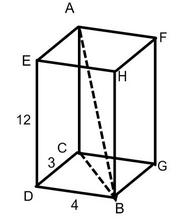# Height of the cuboid

Cuboid with a rectangular base, measuring 3 cm and 4 cm diagonal has a body 13 centimeters long. What is the height of the cuboid?

Correct result:

c =  12 cm

#### Solution:

$a=3 \ \text{cm} \ \\ b=4 \ \text{cm} \ \\ u=13 \ \text{cm} \ \\ \ \\ s^2=a^2 + b^2 \ \\ s=\sqrt{ a^2+b^2 }=\sqrt{ 3^2+4^2 }=5 \ \text{cm} \ \\ \ \\ u^2=c^2 + s^2 \ \\ \ \\ c=\sqrt{ u^2-s^2 }=\sqrt{ 13^2-5^2 }=12 \ \text{cm}$Our examples were largely sent or created by pupils and students themselves. Therefore, we would be pleased if you could send us any errors you found, spelling mistakes, or rephasing the example. Thank you!

Please write to us with your comment on the math problem or ask something. Thank you for helping each other - students, teachers, parents, and problem authors.Tips to related online calculators
Pythagorean theorem is the base for the right triangle calculator.

#### You need to know the following knowledge to solve this word math problem:

We encourage you to watch this tutorial video on this math problem:

## Next similar math problems:

• The regularThe regular triangular prism has a base in the shape of an isosceles triangle with a base of 86 mm and 6.4 cm arms, the height of the prism is 24 cm. Calculate its volume.A regular quadrilateral pyramid has a volume of 24 dm3 and a base edge a = 4 dm. Calculate: a/height of the pyramid b/sidewall height c/surface of the pyramid
• Triangular prismThe triangular prism has a base in the shape of a right triangle, the legs of which is 9 cm and 40 cm long. The height of the prism is 20 cm. What is its volume cm3? And the surface cm2?
• Hexagonal pyramidFind the area of a shell of the regular hexagonal pyramid, if you know that its base edge is 5 cm long and the height of this pyramid is 10 cm.
• Truncated cone 6Calculate the volume of the truncated cone whose bases consist of an inscribed circle and a circle circumscribed to the opposite sides of the cube with the edge length a=1.
• Triangle in a squareIn a square ABCD with side a = 6 cm, point E is the center of side AB and point F is the center of side BC. Calculate the size of all angles of the triangle DEF and the lengths of its sides.
• Integer sidesA right triangle with an integer length of two sides has one leg √11 long. How much is its longest side?
• SailboatThe 20 m long sailboat has an 8 m high mast in the middle of the deck. The top of the mast is fixed to the bow and stern with a steel cable. Determine how much cable is needed to secure the mast and what angle the cable will make with the ship's deck.
• Trip with compassDuring the trip, Peter went 5 km straight north from the cottage, then 12 km west and finally returned straight to the cottage. How many kilometers did Peter cover during the whole trip?
• Right angleIn a right triangle ABC with a right angle at the apex C, we know the side length AB = 24 cm and the angle at the vertex B = 71°. Calculate the length of the legs of the triangle.
• Hexagonal pyramidFind the volume of a regular hexagonal pyramid, the base edge of which is 12 cm long and the side edge 20 cm.
• Regular hexagonal prismCalculate the volume of a regular hexagonal prism whose body diagonals are 24cm and 25cm long.
• Find the 13Find the equation of the circle inscribed in the rhombus ABCD where A[1, -2], B[8, -3] and C[9, 4].
• Calculate 6Calculate the distance of a point A[0, 2] from a line passing through points B[9, 5] and C[1, -1].
• On a lineOn a line p : 3 x - 4 y - 3 = 0, determine the point C equidistant from points A[4, 4] and B[7, 1].
• An observerAn observer standing west of the tower sees its top at an altitude angle of 45 degrees. After moving 50 meters to the south, he sees its top at an altitude angle of 30 degrees. How tall is the tower?
• SailingSolve the following problem graphically. The fishing boat left the harbor early in the morning and set out to the north. After 12 km of sailing, she changed course and continued 9 km west. Then When she docked and reached the fishing grounds she launched